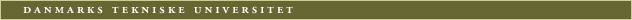Previous page | Current version Archive 1997/1998

 04040 Introductory Statistics and Probability Danish title: Introduktion til statistik og sandsynlighedsregning Type: Å, Language: DDD Credit points: 7,5 point
Previous course: C0410
Offered by: Department of Mathematical Modelling (IMM)
No credit points with: 04041, C0401, C0410
Recommended semester: 1st - 4th semester
Scope and form: 3 lecture modules and 2 hours exercises per week.
Examination: Written exam (13 point scale )
Remarks: The course is a gereral methodological course aimed at all engineering students.
 Contact person: Knut Conradsen, IMM, Building 321, Tel. +45 4525 3416
Aim: To give an introduction to the basic probability theory and a profound understanding of statistical distributions. Furthermore it is the aim to give the participants an introduction to statistical thinking and methods so that they are able to draw up and verify a statistical model for the outcome of (simple) experiments, to estimate unknown parameters in these models (estimation theory), and to examine if a given dataset differs (significantly) from models with parameter values given in advance (test theory). This includes that the participants will learn to analyze contingency tables, to compare the influence of different experimental conditions on measurements (analysis of variance) and to evaluate simple linear relationships (regression analysis).
Contents: Basic probability theory, random variables, multidimensional random variables. Methods to get familiarized with and to process a dataset: Empirical distributions, bar charts and percentile charts. Model formulation. Estimation theory: Properties of the estimators, maximum likelihood, least squares and moment estimators. Test theory: In one and two sample situations. In contingency tables, with distribution free methods. Model control: Test for randomness, control of distribution law. One-way and two-way analysis of variance, Latin squares, regression analysis etc.